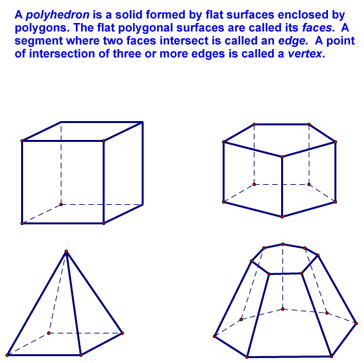you are here > Class Notes - Chapter 12 - Lesson 12-1Printable version

Surface Areas of Prisms - Lesson 12-1

Today we began to discuss Surface Area. In order to do this, we started by discussing polyhedra:We then noted that polyhedra are classified by the number of faces. For instance, a 4-faced polyhedrdon is called a tetrahedron:A 6-faced polyhedron is called a hexahedron:A 7-faced polyhedron is called a heptahedron:And a 10-faced polyhedron is called a decahedron:We then defined a regular polyhedron and showed an example regular dodecahedron:At this point, we defined a prism and learned what the lateral surface area and the total surface area of these polyhedra were:Finally, we defined right prism, oblique prism, and altitude of a prism:At this point, we went through the following examples. First we looked at finding the total surface area of a right rectangular prism:We then looked at this problem - find the total surface area of the right pentagonal prism shown below:In order to solve this problem, we looked at the bases and the lateral faces:These are shapes we know, so we found their area and added them together to get the answer:Other LinksClass NotesLesson 12-1Lesson 12-2Lesson 12-3Quiz TopicsLesson 12-4Lesson 12-5Lesson 12-6Test TopicsIf you have questions, email me at baroodyj@doversherborn.org